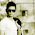## Tuesday, 9 August 2016

### How To Construct SLR(1) Parsing Table

In this post, we will see how to construct SLR(1) parsing table for given grammar.
SLR(1) is Bottom Up Parsing algorithm.

What is meaning of name SLR(1)???
S: Simple
L: Left To Right Scanning of String
R: Reverse to Right Most Derivation
(1): Size of Look ahead is 1. This means one symbol is considered at a time.

Difference between LR(0) and SLR(1):
In LR(0), for a closure which has item of the form (A->BCD.) i.e. item which has Dot at the end, we have to add Reduce action for all terminals. That is why, it is also called to have zero Lookahead symbol.
While in SLR(1), for a closure which has item of the form (A->BCD.) i.e. item which has Dot at the end, we have to add Reduce action for FOLLOW(A) terminals. That is the only difference between LR(0) and SLR(1).

Example: Find the SLR(1) parsing table for the following grammar.

S->AA

A->aA/b

Solution:
For SLR(1) parsing table, we need to find FOLLOW sets of grammar.

FOLLOW(S)={\$}
FOLLOW(A)={a,b,\$}

Note:
1. Remember A->BCD is called a production while A->.BCD or A->B.CD or A->BC.D or A->BCD. are called as "items". The difference between production and item is the placement of new symbol '.' (Dot). Now, what is the significance of Dot? Dot indicates, LHS of Dot is visited while RHS of Dot is still remained to get visited.

2. We have to find items set called as Closure. Closure indicates a state. We move from one closure to another closure after reading a Terminal or a Non-Terminal.

3. We will name these closures as I0, I1,I2..... Here I indicates "Items set" or simply a "closure".

Finding Closure and goto:
Method:
1. Now we will see how to find a closure:
Let us find first closure i.e. I0.
In I0, add item (S'->.S). This indicates we have not read S till now. As Dot is before S, we need productions to evaluate S. Add the items for S i.e. (S->.AA).
In item (S->.AA), Dot is followed by A. Hence again we have to add items for A i.e. (A->.aA) and (A->.b).
In item (A->.aA/.b), Dot is followed by terminals a and b. So no need to add any other item.

2. Now let us see how to find goto:
For each symbol (terminal or non-terminal) which follows Dot, we have add goto (i.e. edge) and we have to find the closures in the similar way.

Check following Diagram of Closures and Goto.Dia. Closure and Goto
In above example, I0,I1,I2,I3 are closures and edges are the "goto" moves.

Note: In above diagram, from I3, for 'a', we get the same state/closure. Hence loop is shown there for goto move 'a'.

Constructing SLR(1) parsing table:
We have to use above Diagram of Closures and Goto.
Note:
1. Rows will have Closure numbers(e.g. 0 for I0, 1 for I1 etc.) while column have Action (for terminals) and Goto (for Non-Terminals).

2. Action part involves two actions Shift and Reduce while Goto involves just a transition from one Closure to another Closure.

3. Check into following parsing table:
In s3, s4.... 's' stands for Shift action while '3' or '4' stands for Closure number.
In r3, r2, r1... 'r' stands for Reduce action while '3' or '2' or '1' stands for production number. In this example. we have numbered productions as follows:
1. S->AA
2. A->aA
3. A->b

Method:
1. For a closure,
For each transition(edge) with terminal, add Si into the table where 'S' represents Shift action and 'i' represents Destination Closure number.
While for each transition(edge) with Non-Terminal, add just a Destination Closure number into Goto part of table.

2. For the closures, which includes items like (A->BCD.) i.e. where Dot is at the end of item, we have to add entry ri where 'r' stands for Reduce action and i stands for production number.
For SLR(1) parsing table, add ri into FOLLOW(A) entries of action part of table.

3. There is a special entry for row 1 and column \$ i.e. "Accept".

SLR(1) Parsing Table:

ClosuresActionGoto
ab\$AS
0s3s421
1Accept
2s3s45
3s3s46
4r3r3r3
5

r1
6r2r2r2

Note: If SLR(1) parsing table contains two entries in any table cell like (S,R) or (R,R), then the grammar is not SLR(1).

1.1.2.